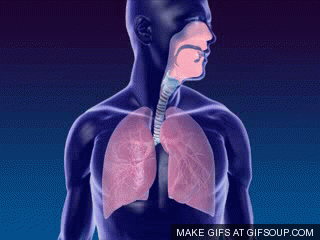# Boyle’s Law Formula And It’s Examples

Boyle’s Law (Constant Temperature) is one of the three special cases of Ideal Gas Law; the other two are Charle’s Law (Constant Pressure) and Gay-Lussac’s Law (Constant Volume). When this law is substituted with Charles’s Law and Gay-Lussac’s Law, develops into Combined Gas Law; further combined with Avogadro’s Law yields Ideal Gas Law.

## Boyle’s Law Formula

When the temperature of a gas is held constant, the pressure of the gas will be inversely proportional to the volume of the sample gas. This phenomenon was discovered by Anglo-Irish Chemist, Inventor, and Physicist Robert Boyle; that is why known as Boyle’s Law.An animation showing the relationship between pressure and volume when amount and temperature held constant Credit: Wikimedia Commons

Mathematically, Boyle’s Law is expressed asorwhere,

P = pressure of the gas
V = volume of the gas
k = constant

Let us compare the same substance under two different conditions, then Boyle’s law can be stated asThe above equation shows that when pressure increases the volume of the gas decreases in proportion.

## Boyle’s Law Examples

Well, examples of Boyle’s Law can be classified into two categories such as Mathematical Examples Of Boyle’s Law and Real-Life Examples Of Boyle’s Law. Let us describe both one by one.

### Mathematical Examples of Boyle’s Law

1. A 5 Litre volume of a gas is at a pressure of 25 atm. A valve allows the gas to flow into a 15 Litre container, connecting two containers. What will be the final pressure of this gas?
V15 Litre
p= ?

ANS = as we know; According to Boyle’s Law Expression

p1 x V1 = p2 x V2

where,

p125 atm
V5 Litre + 15 Litre = 20 Litre
now putting all the values in above equation we get,

p1 x V1 = p2 x V2

25  x 5 =  p2 x 20

on solving,

Final Pressure pwill be 6.25 atm.

2. A balloon with a volume of 3.5 L is filled with a gas at 5 atmospheres. If the pressure of the balloon is reduced to2.5 atmospheres at a constant temperature. What will be the final volume of the balloon?

ANS = as we know; According to Boyle’s Law Expression

p1 x V1 = p2 x V2

where,

p1= 5 atm

p2 = 2.5 atm
V 3.5 Litre

V2 = ?
now putting all the values in above equation we get,

p1 x V1 = p2 x V2

x 3.5 =  2.5 x V2

on solving,

Final Volume Vwill be 7 Litre.

## Examples Of Boyle’s Law In Real Life

If you think, you can’t relate day to day life situations with Boyle’s Law, You Are Wrong. Well, here is your chance to think again!!

There are several examples of Boyle’s Law In Real Life which is easy to understand or even you can do experiments related to them. let’s discuss a few of them one by one.

### The Mechanics Of Human Breathing

I hope we all are aware of what is the mechanics of human breathing !!!! The mechanics of human breathing strictly follows the Boyles law that states that “pressure is inversely proportional to volume“. As we all know the mechanics of breathing involve two different processes i.e Exhale and Inhale.The Mechanics Of Human Breathing (Inhaling And Exhaling) Credit: Giphy

When we inhale, the volume of lung increases which in turn decreases the pressure inside the lungs compared to the outer atmosphere, and as a result air rushes inside the lungs. On the other hand, when we exhale, the volume of the lungs decreases which in turn increases the pressure inside the lungs compared to the outer atmosphere, and as a result air rushes outside of the lungs. Well, if you don’t believe me, check your self.

### Storage Of Gases

Nowadays almost each and every industry is somehow using Boyle’s Law for the storage of gases. In order to store gas in a small container, gases are highly pressurized, resulting in the reduction of the volume of the gas. For example, like the deodorants we use or like the most common one is the LPG(Liquefied Petroleum Gas) gas container we use for cooking purposes.